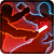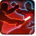# Unknowing RageConditions

## Used by

Triggered by equipping an item (1)

## Related effects, buffs and debuffs

• [hidden] [does not expire]Effect #1

 Slot: Buff Duration: passive Tick rate: does not tick # occurrences: 1
• After an ability is activated by NONE

Perform the following actions:

• Unknown (193)
- Unknown (601) = (bool) false
- Unknown (602) = (bool) false
- Unknown (607) = (bool) false
- Tags = (string)
- Ability Spec = (int) 16140901905952099253
- Amount Max = (float) 1
- Amount Min = (float) 1
- Amount Percent = (float) 0
• After an ability is activated by NONE

Perform the following actions:

• Unknown (193)
- Unknown (601) = (bool) false
- Unknown (602) = (bool) false
- Unknown (607) = (bool) false
- Tags = (string)
- Ability Spec = (int) 16140913435762888184
- Amount Max = (float) 1
- Amount Min = (float) 1
- Amount Percent = (float) 0